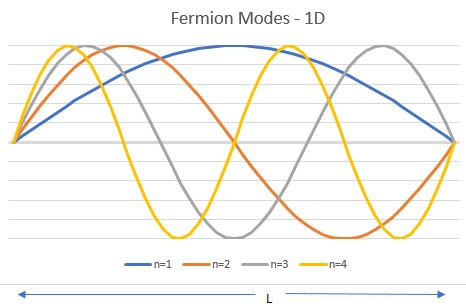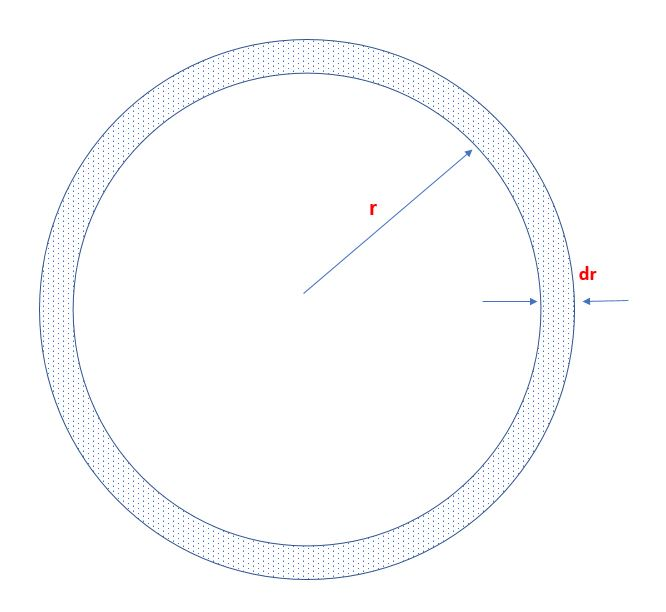# Fermi Gases and Stellar Collapse – Cosmology Post #6

The most refined Standard Candle there is today is a particular kind of Stellar Collapse, called a Type 1a Supernova. To understand this, you will need to read the previous posts (#1-#5), in particular, the Fermi-Dirac statistics argument in Post #5 in the sequence. While this is the most mathematical of the posts, it might be useful to skim over the argument to understand the reason for the amazing regularity in these explosions.

Type 1a supernovas happen to white dwarf stars. A white dwarf is a kind of star that has reached the end of its starry career. It has burnt through its hydrogen fuel, producing all sorts of heavier elements, through to carbon and oxygen. It has also ceased being hot enough to burn Carbon and Oxygen in fusion reactions. Since these two elements burn rather less efficiently than Hydrogen or Helium in fusion reactions, the star is dense (there is less pressure from light being radiated by fusion reactions in the inside to counteract the gravitational pressure of matter, so it compresses itself) and the interior is composed of ionized carbon and oxygen (all the negatively charged electrons are pulled out of every atom, the remaining ions are positively charged and the electrons roam freely in the star). Just as in a crystalline lattice (as in a typical metal), the light electrons are good at keeping the positively charged ions screened from other ions. In addition, they also use them in turn to screen themselves from other electrons; the upshot is, the electrons behave like free particles.

At this point, the star is being pulled in by its own mass and is being held up by the pressure exerted by the gas of free electrons in its midst. The “lattice” of positive ions also exerts pressure, but the pressure is much less, as we will see. The temperature of the surface of the white dwarf is known from observations to be quite high,$\sim 10,000-100,000 \:$ Kelvin. More important, the free electrons in a white dwarf of mass much greater than the Sun’s mass (written as$M_{\odot}$) are ultra-relativistic, with energy much higher than their individual mass. Remember, too, that electrons are a species of “fermion”, which obey Fermi-Dirac statistics.

The Fermi-Dirac formula is written as$P(\vec k) = 2 \frac {1}{e^{\frac{\hbar c k - \hbar c k_F}{k_B T}}+1}$

What does this formula mean? The energy of an ultra-relativistic electron, that has energy far in excess of its mass, is$E = \hbar c k$

where$k c$ is the “frequency” corresponding to the electron of momentum$c k$, while$\hbar$ is the “reduced” Planck’s constant$(=\frac {h}{2 \pi})$ (here$h$ is the regular Planck’s constant) and$c$ is the speed of light. The quantity$k_F$ is called the Fermi wave-vector.  The function$P(\vec k)$ is the (density of) probability of finding an electron in the momentum state specified by$\hbar \vec k$. In the study of particles where their wave nature is apparent, it is useful to use the concept of the de Broglie “frequency”$(\nu = \frac{E}{h})$, the de Broglie “wavelength”$(\lambda=\frac {V}{\nu}$ where$V$ is the particle velocity) and$k=\frac{2 \pi}{\lambda}$, the “wave-number”  corresponding to the particle. It is customary for lazy people to forget to write$c$ and$\hbar$ in formulas, hence, we speak of momentum$k$ for a hyper-relativistic particle travelling at speed close to the speed of light, when it should really be$h \frac {\nu}{c} = h \frac{V}{\lambda c} \approx {h}{\lambda} = \frac {h}{2 \pi} \frac{2 \pi}{\lambda} = {\bf {\hbar k}}$.

Why a factor of 2? It wasn’t there in the previous post!

From the previous post, you know that fermions don’t like to be in the same state together. We also know that electrons have a property called spin and they can be spin-up or spin-down. Spin is a property akin to angular momentum, which is a property that we understand classically, for instance, as describing the rotation of a bicycle wheel. You might remember that angular momentum is conserved unless someone applies a torque to the wheel. This is the reason why free-standing gyroscopes can be used for airplane navigation – they “remember” which direction they are pointing in. Similarly, spin is usually conserved, unless you apply a magnetic field to “twist” a spin-up electron into a spin-down configuration. So, you can actually have two kinds of electrons – spin-up and spin-down, in each momentum state$\vec k$. This is the reason for the factor of 2 in the formula above – there are two “spin” states per$\vec k$ state.

Let’s understand the Fermi wave-vector$k_F$. Since the fermions need to occupy momentum states two-at-a-time for each, and if they were forced into a cube of side$L$, you can ask how many levels they occupy. They will, like all sensible particles, start occupying levels starting with the lowest energy level, going up till all the fermions available are exhausted. The fermions are described by waves and, in turn, waves are described by wavelength. You need to classify all the possible ways to fit waves into a cube. Let’s look at a one-dimensional case to startThe fermions need to bounce off the ends of the one-dimensional lattice of length$L$ so we need the waves to be pinned to 0 at the ends. If you look at the above pictures, the wavelengths of the waves are$2L$ (for n=1),$L$ (for n=2),$\frac{2 L}{3}$ (for n=3),$\frac {L}{2}$ (for n=4).  In that case, the wavenumbers, which are basically$\frac {2 \pi}{\lambda}$ for the fermions need to be of the sort$\frac {n \pi}{L}$, where$n$ is an integer$(0, \pm 1, \pm 2 ...)$.For a cube of side L, the corresponding wave-numbers are described by$\vec k = (n_x, n_y, n_z) \frac {\pi}{L}$ since a vector will have three components in three dimensions. These wave-numbers correspond to the momenta of the fermions (this is basically what’s referred to as wave-particle duality), so the momentum is$\vec p = \hbar \vec k$. The energy of each level is$\hbar c k$. It is therefore convenient to think of the electrons as filling spots in the space of$k_x, k_y, k_z$.

What do we have so far? These “free” electrons are going to occupy energy levels starting from the lowest$k = (\pm 1, \pm 1, \pm 1) \frac{\pi}{L}$ and so on in a neat symmetric fashion. In$k$ space, which is “momentum space”, since we have many, many electrons, we could think of them filling up a sphere of radius$k_F$ in momentum space. This radius is called the Fermi wave-vector. It represents the most energetic of the electrons, when they are all arranged in as economically as possible – with the lowest possible energy for the gas of electrons. This would happen at zero temperature (which is the approximation we are going to work with at this time). This comes out from the probability distribution formula (ignore the 2 for this, consider the probability of occupation of levels for a one-dimensional fermion gas). Note that all the electrons are inside the Fermi sphere at low temperature and leak out as the temperature is raised (graphs towards the right).

It is remarkable and you should realize this, that a gas of fermions in its lowest energy configuration has a huge amount of energy. The Pauli principle requires it. If they were bosons, all of them would be sitting at the lowest possible energy level, which couldn’t be zero (because we live in a quantum world) but just above it.

What’s the energy of this gas? Its an exercise in arithmetic for zero temperature. Is that good enough? No, but it gets us pretty close to the correct answer and it is instructive.

The total energy in the gas of electrons is (in a spherical white dwarf of volume$V = \frac {4}{3} \pi R^3$, with$2$ spins per state, is$E_{Total} = 2 V \int \frac {d^3 \vec k}{(2 \pi)^3} \hbar c k = V \frac {\hbar c k_F^4}{4 \pi^2}$

The total number of electrons is obtained by just adding up all the available states in momentum space, up to$k_F$$N = 2 V \int \frac {d^3 \vec k}{(2 \pi)^3} \rightarrow k_F^3 = 3 \pi^2 \frac {N}{V}$

We need to estimate the number of electrons in the white dwarf to start this calculation off. That’s what sets the value of$k_F$, the radius of the “sphere” in momentum space of filled energy states at zero temperature.

The mass of the star is$M$. That corresponds to$\frac {M} { \mu_e m_p}$ full atoms, where$m_p$ is the mass of the proton (the dominant part of mass) and$\mu_e$ is the ratio of atomic weight to atomic number for a typical constituent atom in the white dwarf. For a star composed of Carbon and Oxygen, this is$2$. So,$N = V \frac {M}{\mu_e m_p} = V \frac {M}{2 m_p}$.

Using all the above$E_{Total} = \frac {4\pi}{3} R^3 \frac {\hbar c}{4 \pi^2} \left( 3 \pi^2 \frac {M}{\mu_e m_p \frac{4\pi}{3} R^3 }\right)^{4/3}$

Next, the white dwarf has some gravitational potential energy just because of its existence. This is calculated in high school classes by integration over successive spherical shells from$0$ to the radius$R$, as shown belowThe gravitational potential energy is$\int_{0}^{R} (-) G \frac {\frac{4 \pi}{3} \rho_m r^3 4 \pi r^2}{r} dr = - \frac{3}{5} \frac {G M^2}{R}$

A strange set of things happen if the energy of the electrons (which is called, by the way, the “degeneracy energy”) plus the gravitational energy goes negative. At that point, the total energy can become even more negative as the white dwarf’s radius gets smaller – this can continue${\it ad \: infinitum}$ – the star collapses. This starts to  happen when you set the gravitational potential energy equal to the Fermi gas energy, this leads to$\frac{3}{5} \frac{G M^2}{R} = \frac {4\pi}{3} R^3 \frac {\hbar c}{4 \pi^2} \left( 3 \pi^2 \frac {M}{\mu_e m_p \frac{4\pi}{3} R^3 }\right)^{4/3}$

the$R$ (radius) of the star drops out and we are left with a unique mass$M$ where this happens – the calculation above gives an answer of$1.7 M_{\odot}$. A more precise calculation at non-zero temperature gives$1.44 M_{\odot}$.

The famous physicist S. Chandrasekhar, after whom the Chandra X-ray space observatory is named, discovered this (Chandrasekhar limit) while ruminating about the effect of hyper-relativistic fermions in a white dwarf.He was on  a cruise from India to Great Britain at the time and had the time for unrestricted rumination of these sorts!

Therefore, as is often the case, if a white dwarf is surrounded by a swirling cloud of gas of various sorts or has a companion star of some sort that it accretes matter from, it will collapse into a denser neutron star in a cataclysmic collapse precisely when this limit is reached. If so, once one understands the type of light emitted from such a supernova from some nearer location, one has a Standard Candle – it is like having a hand grenade that is of${\bf exactly}$ the same quality at various distances. By looking at how bright the explosion is, you can tell how far away it is.

After this longish post, I will describe the wonderful results from this analysis in the next post – it has changed our views of the Universe and our place in it, in the last several years.